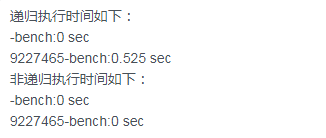# 【web 开发基础】PHP 中的递归函数 (38)

• 2022-11-28
广东
• 本文字数：1356 字

阅读完需：约 4 分钟

## 编程实践

<?php//使用递归方式求斐波那契数function fb($n){  if($n <=2){    return 1;  }else{    return fb($n-1) + fb($n-2);  }}echo fb(8);<?phpfunction fb($n){  if($n <=2){    return 1;  }  $t1 = 1;$t2 = 1;  for($i=3;$i<$n;$i++){    $temp =$t1;    $t1 =$t2;    $t2 =$temp + $t2; } return$t1 + \$t2;}echo fb(8);## 评论